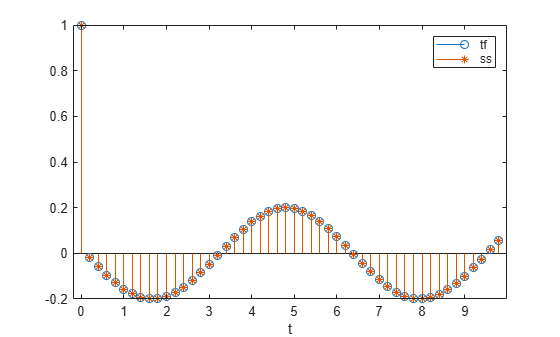Documentation

# tf2ss

Convert transfer function filter parameters to state-space form

## Syntax

``[A,B,C,D] = tf2ss(b,a)``

## Description

example

````[A,B,C,D] = tf2ss(b,a)` converts a continuous-time or discrete-time single-input transfer function into an equivalent state-space representation.```

## Examples

collapse all

Consider the system described by the transfer function

`$H\left(s\right)=\frac{\left[\begin{array}{c}2s+3\\ {s}^{2}+2s+1\end{array}\right]}{{s}^{2}+0.4s+1}.$`

Convert it to state-space form using `tf2ss`.

```b = [0 2 3; 1 2 1]; a = [1 0.4 1]; [A,B,C,D] = tf2ss(b,a)```
```A = 2×2 -0.4000 -1.0000 1.0000 0 ```
```B = 2×1 1 0 ```
```C = 2×2 2.0000 3.0000 1.6000 0 ```
```D = 2×1 0 1 ```

A one-dimensional discrete-time oscillating system consists of a unit mass, $\mathit{m}$, attached to a wall by a spring of unit elastic constant. A sensor samples the acceleration, $\mathit{a}$, of the mass at ${\mathit{F}}_{\mathrm{s}}=5$ Hz.Generate 50 time samples. Define the sampling interval $\Delta \mathit{t}=1/{\mathit{F}}_{\mathrm{s}}$.

```Fs = 5; dt = 1/Fs; N = 50; t = dt*(0:N-1); u = [1 zeros(1,N-1)];```

The transfer function of the system has an analytic expression:

$\mathit{H}\left(\mathit{z}\right)=\frac{1-{\mathit{z}}^{-1}\left(1+\mathrm{cos}\Delta \mathit{t}\right)+{\mathit{z}}^{-2}\mathrm{cos}\Delta \mathit{t}}{1-2{\mathit{z}}^{-1}\mathrm{cos}\Delta \mathit{t}+{\mathit{z}}^{-2}}$.

The system is excited with a unit impulse in the positive direction. Compute the time evolution of the system using the transfer function. Plot the response.

```bf = [1 -(1+cos(dt)) cos(dt)]; af = [1 -2*cos(dt) 1]; yf = filter(bf,af,u); stem(t,yf,'o') xlabel('t')```Find the state-space representation of the system. Compute the time evolution starting from an all-zero initial state. Compare it to the transfer function prediction.

```[A,B,C,D] = tf2ss(bf,af); x = [0;0]; for k = 1:N y(k) = C*x + D*u(k); x = A*x + B*u(k); end hold on stem(t,y,'*') hold off legend('tf','ss')```## Input Arguments

collapse all

Transfer function numerator coefficients, specified as a vector or matrix. If `b` is a matrix, then each row of `b` corresponds to an output of the system.

• For discrete-time systems, `b` contains the coefficients in descending powers of z.

• For continuous-time systems, `b` contains the coefficients in descending powers of s.

For discrete-time systems, `b` must have a number of columns equal to the length of `a`. If the numbers differ, make them equal by padding zeros. You can use the function `eqtflength` to accomplish this.

Transfer function denominator coefficients, specified as a vector.

• For discrete-time systems, `a` contains the coefficients in descending powers of z.

• For continuous-time systems, `a` contains the coefficients in descending powers of s.

## Output Arguments

collapse all

State matrix, returned as a matrix. If the system is described by n state variables, then `A` is n-by-n.

Data Types: `single` | `double`

Input-to-state matrix, returned as a matrix. If the system is described by n state variables, then `B` is n-by-1.

Data Types: `single` | `double`

State-to-output matrix, returned as a matrix. If the system has q outputs and is described by n state variables, then `C` is q-by-n.

Data Types: `single` | `double`

Feedthrough matrix, returned as a matrix. If the system has q outputs, then `D` is q-by-1.

Data Types: `single` | `double`

collapse all

### Transfer Function

`tf2ss` converts the parameters of a transfer function representation of a given system to those of an equivalent state-space representation.

• For discrete-time systems, the state-space matrices relate the state vector x, the input u, and the output y:

`$\begin{array}{c}x\left(k+1\right)=Ax\left(k\right)+Bu\left(k\right),\\ y\left(k\right)=Cx\left(k\right)+Du\left(k\right).\end{array}$`

The transfer function is the Z-transform of the system’s impulse response. It can be expressed in terms of the state-space matrices as

`$H\left(z\right)=C{\left(zI-A\right)}^{-1}B+D.$`

• For continuous-time systems, the state-space matrices relate the state vector x, the input u, and the output y:

`$\begin{array}{l}\stackrel{˙}{x}=Ax+Bu,\\ y=Cx+Du.\end{array}$`

The transfer function is the Laplace transform of the system’s impulse response. It can be expressed in terms of the state-space matrices as

`$H\left(s\right)=\frac{B\left(s\right)}{A\left(s\right)}=\frac{{b}_{1}{s}^{n-1}+\cdots +{b}_{n-1}s+{b}_{n}}{{a}_{1}{s}^{m-1}+\cdots +{a}_{m-1}s+{a}_{m}}=C{\left(sI-A\right)}^{-1}B+D.$`